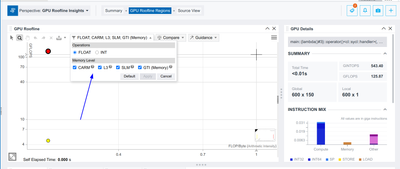Analyzers
Support for Analyzers (Intel VTune™ Profiler, Intel Advisor, Intel Inspector)
4751 Discussions

## No gpu info was collected with advisor -collect rooflineNovice
291 Views

Here is a program of matrix-matrix multiplication. I can not collect any gpu info with the command:

Here is the result:I want to collect the GFLOPS of gemm_micro_kernel, can any one help me?

Versions:

Intel(R) Advisor 2022.1 (build 613050) Command Line Tool

Ubuntu 20.04

Intel(R) oneAPI DPC++/C++ Compiler 2022.1.0 (2022.1.0.20220316)

GPU: ATS-P

How to compile:

Code:

``````#include <iostream>
#include <string>
#include <cstring>
#include <CL/sycl.hpp>
#include <chrono>
#include <random>

#define bm 16
#define bn 16
#define bk 16
#define upper_divide(a, b) ((((a) - 1) / (b) + 1) * (b))

using kernel_type = float;
sycl::queue q(sycl::gpu_selector{});

constexpr size_t m = 100, n = 200, k = 300;

void demo_data(kernel_type* amat, kernel_type* bmat, kernel_type* result);
void gemm_micro_kernel(kernel_type* A, kernel_type* B, kernel_type* C); // kernel5 + kernel3

int main(int argc, char** argv)
{
kernel_type* amat = new kernel_type[m*k];
kernel_type* bmat = new kernel_type[k*n];
kernel_type* result = new kernel_type[m*n];
demo_data(amat, bmat, result);

// init
kernel_type* amat_dev = sycl::malloc_device<kernel_type>(m*k, q);
kernel_type* bmat_dev = sycl::malloc_device<kernel_type>(k*n, q);
q.memcpy(amat_dev, amat, m*k * sizeof(kernel_type)).wait();
q.memcpy(bmat_dev, bmat, k*n * sizeof(kernel_type)).wait();
q.parallel_for(sycl::range(m, k), [=](sycl::id<2> it) {
A[it * pad_k + it] = amat_dev[it * k + it];
}).wait();
q.parallel_for(sycl::range(k, n), [=](sycl::id<2> it) {
B[it * pad_n + it] = bmat_dev[it * n + it];
}).wait();
std::cout << "init done.\n";

gemm_micro_kernel(A, B, output);

auto us = std::chrono::duration_cast<std::chrono::microseconds>(t2 - t1).count();
double ms = (double)us / 1000;
std::cout << "kernel time: " << us << " us. i.e " << ms << "ms.\n";

return 0;
}

void gemm_micro_kernel(kernel_type* A, kernel_type* B, kernel_type* C)
{
q.submit([&](sycl::handler& h) {
sycl::accessor<kernel_type, 1, sycl::access::mode::read_write, sycl::access::target::local> sa(sycl::range(bm*4 * bk), h);
sycl::accessor<kernel_type, 1, sycl::access::mode::read_write, sycl::access::target::local> sb(sycl::range(bk * bn), h);
h.parallel_for(sycl::nd_range<2>(global_range, local_range), [=](sycl::nd_item<2> it) [[intel::reqd_sub_group_size(16)]] {
size_t i = it.get_global_id(0) * 4;
size_t j = it.get_global_id(1);
size_t ti = it.get_local_id(0);
size_t tj = it.get_local_id(1);

kernel_type sum_arr = {0, 0, 0, 0};
kernel_type b00;
for (size_t ki = 0; ki < pad_k; ki += bk) {
sa[(ti*4)*bk   + tj] = A[i*pad_k     + ki + tj];
sa[(ti*4+1)*bk + tj] = A[(i+1)*pad_k + ki + tj];
sa[(ti*4+2)*bk + tj] = A[(i+2)*pad_k + ki + tj];
sa[(ti*4+3)*bk + tj] = A[(i+3)*pad_k + ki + tj];

sb[tj*bn + ti  ] = B[(ki+ti)*pad_n   + j];
it.barrier(sycl::access::fence_space::local_space);
#pragma unroll
for (size_t inner_k_count = 0; inner_k_count < bk; inner_k_count++) {
b00 = sb[tj * bn + inner_k_count];
sum_arr += sa[(ti*4) * bk + inner_k_count] * b00;
sum_arr += sa[(ti*4+1) * bk + inner_k_count] * b00;
sum_arr += sa[(ti*4+2) * bk + inner_k_count] * b00;
sum_arr += sa[(ti*4+3) * bk + inner_k_count] * b00;
}
it.barrier(sycl::access::fence_space::local_space);
}
C[i * pad_n + j] = sum_arr;
C[(i+1) * pad_n + j] = sum_arr;
C[(i+2) * pad_n + j] = sum_arr;
C[(i+3) * pad_n + j] = sum_arr;
});
});
q.wait();
}

void demo_data(kernel_type* amat, kernel_type* bmat, kernel_type* result)
{
size_t id = 0;
std::default_random_engine e(time(0));
std::uniform_real_distribution<double> u(-100, 100);
for (size_t i = 0; i < m*k; ++i) amat[i] = u(e);
for (size_t i = 0; i < k*n; ++i) bmat[i] = u(e);
}
``````

Labels (1)

1 SolutionModerator
135 Views

Hi,

We are able to get GPU Roofline chart using your workload. However, we have increased workload size to make it x100 time bigger.

Please find the attached report containing GPU info .

Hope this addresses your queries. If so, kindly click the “Accept as Solution” button to indicate that your issue is resolved. This will also help others with a similar issue.

Please let us know if we can close the case.

Thanks

7 RepliesModerator
255 Views

Hi,

Thank you for posting in Intel communities.

Sorry for the delay. We are able to reproduce the issue from our end and we could see that the reason behind the low execution time is your code. Since your code takes very small time to execute it is not get captured.

If this clarify your query, make sure to accept this as a solution. This would help others with similar issues.

ThanksNovice
241 Views

But advisor works in this kernel with same size of inputs

``````    q.submit([&](sycl::handler& h) {
h.parallel_for(sycl::nd_range<2>(global_range, local_range), [=](sycl::nd_item<2> it) {
size_t i = it.get_global_id(0);
size_t j = it.get_global_id(1);
kernel_type sum = 0;
for (size_t ki = 0; ki < k; ++ki) {
sum += A[i * pad_k + ki] * B[ki * pad_n + j];
}
C[i * pad_n + j] = sum;
});
}).wait();``````

Actually, even I input a huge sample to the previous kernel, advisor still can not capture any flops.Moderator
196 Views

Hi,

Could you please try to run the same analysis with the matrix_mul_dpcpp.cpp which is available in the below GitHub link.

https://github.com/oneapi-src/oneAPI-samples/tree/2022.3/DirectProgramming/DPC%2B%2B/DenseLinearAlge...

Please share the screenshot to verify whether gpu info is collected or not.

ThanksNovice
165 Views

here is the `summary` tabbut I can see the result in `GPU Roofline Regions` tab with this options checkedBTW, here is the CLI outputModerator
136 Views

Hi,

We are able to get GPU Roofline chart using your workload. However, we have increased workload size to make it x100 time bigger.

Please find the attached report containing GPU info .

Hope this addresses your queries. If so, kindly click the “Accept as Solution” button to indicate that your issue is resolved. This will also help others with a similar issue.

Please let us know if we can close the case.

ThanksNovice
118 Views

Thank you very much.Moderator
112 Views

Hi,

Thanks for accepting our solution. Glad to know that your issue is resolved.

If you need any additional information, please post a new question as this thread will no longer be monitored by Intel.

Thanks